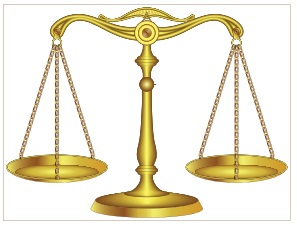Home | | Science 6th Std | Mass

# Mass

Mass is the measure of the amount of matter in an object. Weight is the gravitational pull experienced by the mass.

Mass

1. Mass and Weight

Mass is the measure of the amount of matter in an object. Weight is the gravitational pull experienced by the mass.

Hold a sheet of paper in one hand and a book in another. Which hand feels the heavyness? The mass of the book is more than that of a single sheet of paper. Therefore the pull on the book is more than that of the paper. Hence our hand has to give more force to hold a book than a paper. This force is what we experience as ‘heaviness’.

More to Know

The weight is directly proportional to the mass on earths surface. On moon where the gravitational force is lesser than earth, the weight will reduce but the mass will remain same. The moon’s gravitational pull is one sixth of the earth’s pull. Thus objects weigh six times lighter on the Moon than on the Earth.

The SI unit of mass is kilogram. It is represented by the ‘kg’.

Now a question, what is your mass? If you measure it grams that would be a huge number is it not? So it is expressed in kilogram. Bigger weights are measured in tonne or Metric Tonne.

1000 milligram = 1 gram

1000 gram = 1 kilogram

1000 kilogram = 1 tonne

2. Beam Balance

We use beam balances to measure mass. A beam balance works by comparing the mass of an object to that of known mass (called a standard mass)Activity 5

Construct your own beam balance using two scrapped coconut shells, strings or twines thick cardboard as frame and a little sharpened pencil as index needle.

What can you achieve?

1. Find which object is heavier

2. Find approximate weight of lighter things (leaves, piece of papers, etc)We achieve beam balance model.

Student doing activity.

3. Electronic Balance

An electronic balance is a device used to find accurate measurements of weight. It is used very commonly in laboratories for weighing chemicals to ensure a precise measurement of those chemicals for use in various experiments. Electronic balances may also be used to weigh food, other grocery items, as well as jewellery.Tags : Measurements | Term 1 Unit 1 | 6th Science , 6th Science : Term 1 Unit 1 : Measurements
Study Material, Lecturing Notes, Assignment, Reference, Wiki description explanation, brief detail
6th Science : Term 1 Unit 1 : Measurements : Mass | Measurements | Term 1 Unit 1 | 6th Science